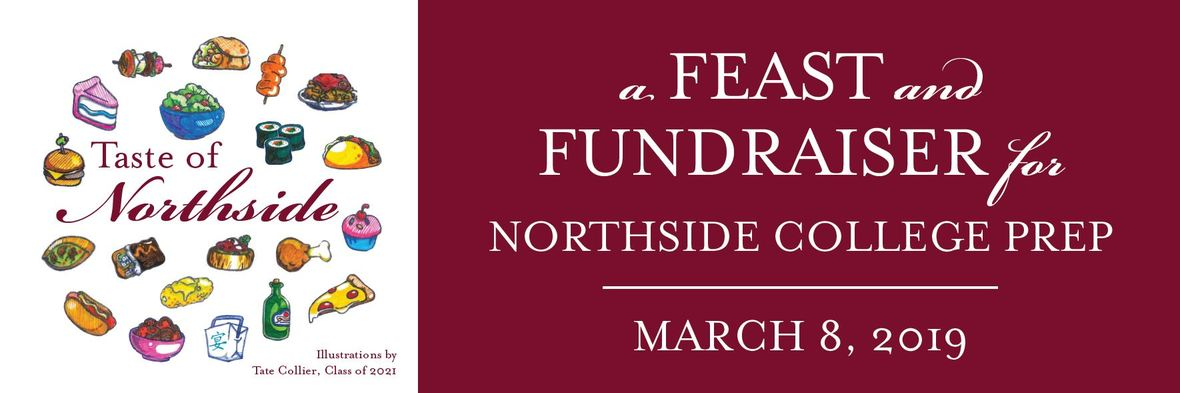# Please Pick Up Your Auction Items

Monday, March 18, 2:00 - 4:00 pm
Tuesday, March 19, 8:00 - 9:00 am
Northside College Prep

We're extremely grateful to everyone who placed a bid, generously donated an auction item or invited family and friends to participate.

If you need to make other arrangements, email Kristen Werries Collier, kristenwerriescollier@gmail.com.

# Fund-a-Need

## The Most Direct Way to Support Learning at Northside

Our teachers have compiled a thoughtful list of their immediate needs. Donate now to ensure that they have the equipment and supplies that they need to offer all of our students the enriched and exceptional curriculum that NCP is known for.
With your help, our students can have:

 ▪ Headsets
 table div table+table+table+table+table+table+table div table{width:100%;padding:0}table div table+table+table+table+table+table+table div table img{width:96.23%;padding:0;float:none}table div table+table+table+table+table+table+table div table td{width:100%;padding:0 1.88% 18px}/* styles */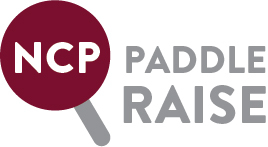# Better Library, Brighter Future!

## The NCP Library: the heart of the Northside community since 1998.

The library is the heart of NCP where students gather to study, collaborate and socialize. Help us to modernize and revitalize the space for our motivated and talented students. Let’s provide our community with a library that meets the needs of our students in 2019 and beyond. Click HERE to hear from freshman Jake Philips and HERE to hear from sophomore Marco Salzetta. Please make a donation to the NCP Fund for the Library today!

 table div table+table+table+table+table+table+table+table+table+table div table{width:100%;padding:0}table div table+table+table+table+table+table+table+table+table+table div table img{width:96.23%;padding:0;float:none}table div table+table+table+table+table+table+table+table+table+table div table td{width:100%;padding:0 1.88% 18px}/* styles */# Parent Parties

Sign up to attend a party hosted by a Northside family. These parties are a great opportunity to meet and mingle with other Northside parents. We have something for everyone. Don't miss out, sign up today!

# Dungeons & Dragons Party

Don't miss this night of RPG adventure and fine tavern fare.
Saturday, May 4 at 6:00 pm.

## Tacos and Tequila!

Celebrate Cinco de Mayo with chips and guacamole, tacos, margaritas and tequila. Sunday May 5 at 4:30 pm.

## Indian Cuisine Dinner Party

Join Northside parents for a night of delicious home made Indian food. Saturday, May 11. Only 1 spot left!

## Trivia Night

Test your trivia knowledge, enjoy some pizza and beverages, socialize with other NCP parents and maybe win a prize!
Thursday, May 16 at 6:30 pm.

# Moms' Margarita Party

Enjoy a night of margaritas, music, and Mexican cuisine.
Friday, May 31.

# Moms Who Brunch

Join other Northside moms for delicious brunch items and cocktails.
Sunday, June 2

# Bourbon, Beers, & Burgers

Don't miss this casual celebratory night of Bourbon, Beers, and Burgers to mark the end of the school year.
Saturday, June 22 at 7:00 pm.

# Evereve Shopping Party

Mom's Night Out Styling Party at Evereve Southport. Delicious wine and appetizers will be served.
Saturday, September 7 at 6:00 pm.

 table div table+table+table+table+table+table+table+table+table+table+table+table+table+table+table+table+table+table+table+table div table{width:100%;padding:0}table div table+table+table+table+table+table+table+table+table+table+table+table+table+table+table+table+table+table+table+table div table img{width:96.23%;padding:0;float:none}table div table+table+table+table+table+table+table+table+table+table+table+table+table+table+table+table+table+table+table+table div table td{width:100%;padding:0 1.88% 18px}/* styles */## Click HERE to see photos from the event.

 table div table+table+table+table+table+table+table+table+table+table+table+table+table+table+table+table+table+table+table+table+table+table div table{width:100%;padding:0}table div table+table+table+table+table+table+table+table+table+table+table+table+table+table+table+table+table+table+table+table+table+table div table img{width:96.23%;padding:0;float:none}table div table+table+table+table+table+table+table+table+table+table+table+table+table+table+table+table+table+table+table+table+table+table div table td{width:100%;padding:0 1.88% 18px}/* styles */# This Week in Sports

Registration for spring sports is now open on the 8to18 website. Please be sure to register your students as soon as possible. Click HERE.

Women's Soccer

▪ Tuesday, March 19 (V and JV) v. Niles West at Niles West
▪ Thursday, March 21 (V and JV) v. Juarez at Juarez
 ▪ Tuesday, March 19 (V and JV) v. Niles West at Niles West
 ▪ Thursday, March 21 (V and JV) v. Juarez at Juarez

Men’s Baseball

▪ Monday, March 18, 4:30 pm (V and SO) v. Washington at Rogers Park
▪ Tuesday, March 19, 4:30 pm (V and SO) v. Maine East at Maine East
▪ Wednesday, March 20, 4:30 pm (SO) v. Parker at Rogers Park
▪ Friday, March 22, 4:30 pm (V and SO) v. Senn at Rogers Park
 ▪ Monday, March 18, 4:30 pm (V and SO) v. Washington at Rogers Park
 ▪ Tuesday, March 19, 4:30 pm (V and SO) v. Maine East at Maine East
 ▪ Wednesday, March 20, 4:30 pm (SO) v. Parker at Rogers Park
 ▪ Friday, March 22, 4:30 pm (V and SO) v. Senn at Rogers Park

Women’s Lacrosse

▪ Wednesday, March 20, 5:00 pm (V) v. Jones at Winnemac Park
▪ Thursday, March 21, 5:15/6:45 pm (JV/V) v. Hoffman Estates at Hoffman Estates High School
 ▪ Wednesday, March 20, 5:00 pm (V) v. Jones at Winnemac Park
 ▪ Thursday, March 21, 5:15/6:45 pm (JV/V) v. Hoffman Estates at Hoffman Estates High School

Men’s Lacrosse

▪ Tuesday, March 19, 5:30 pm (JV) v. Riverside-Brookfield @ Riverside
▪ Wednesday, March 20, 5:00 pm (V) v. Lane Tech @ Lane Tech
 ▪ Tuesday, March 19, 5:30 pm (JV) v. Riverside-Brookfield @ Riverside
 ▪ Wednesday, March 20, 5:00 pm (V) v. Lane Tech @ Lane Tech

Women's Softball

▪ Monday, March 18, 4:30 pm (V/SO) v. Hancock at Home (Devon & Kedzie)
▪ Wednesday, March 20, 4:30 pm (V/SO) v. Ag. Science at Home (Devon & Kedzie)
▪ Thursday, March 21, 4:45 pm (V) v. Niles North at Niles North
▪ Saturday, March 23, 10:00 am (V/SO) v. Maine East at Maine East
 ▪ Monday, March 18, 4:30 pm (V/SO) v. Hancock at Home (Devon & Kedzie)
 ▪ Wednesday, March 20, 4:30 pm (V/SO) v. Ag. Science at Home (Devon & Kedzie)
 ▪ Thursday, March 21, 4:45 pm (V) v. Niles North at Niles North
 ▪ Saturday, March 23, 10:00 am (V/SO) v. Maine East at Maine East

Men’s Volleyball

▪ Tuesday, March 19, 5:00 pm (V) v. Lane Tech at Northside
 ▪ Tuesday, March 19, 5:00 pm (V) v. Lane Tech at Northside

To all parents of freshman and sophomore boys — the NCP baseball program is looking for players for the Frosh/Soph team. If your son is interested in joining the fun as a walk-on, please contact Coach Bill Rosencrans at wrosencrans@cps.edu or ask a baseball player about getting involved.

 table div table+table+table+table+table+table+table+table+table+table+table+table+table+table+table+table+table+table+table+table+table+table+table+table div table{width:100%;padding:0}table div table+table+table+table+table+table+table+table+table+table+table+table+table+table+table+table+table+table+table+table+table+table+table+table div table img{width:96.23%;padding:0;float:none}table div table+table+table+table+table+table+table+table+table+table+table+table+table+table+table+table+table+table+table+table+table+table+table+table div table td{width:100%;padding:0 1.88% 18px}/* styles */# Buy your 2018-2019 Stampede Now!

## Ordering deadline is March 27

The deadline for purchasing the 2018-2019 Stampede is Wednesday, March 27. The price is now \$55.00. After this date, you won’t be guaranteed a yearbook, since we will not be ordering many extra copies.

There was not an option to pay for a yearbook with a school fee, so you need to go to the Lifetouch site.

To purchase a yearbook please click the link below and follow the directions.
1) Go to https://ybpay.lifetouch.com/Order/SelectJob
2) Enter code 4187219# DEADLINE FOR SENIOR ADS IS TOMORROW!

## Purchase Yearbook Personal Ads for Seniors

All materials are due by Monday, March 18.

Commemorate your student's accomplishments or declare your best wishes with a personal ad in the upcoming Stampede. The prices are:

▪ Full Page \$150
▪ Half Page \$80
▪ Quarter Page \$50

Members of the Stampede can create the ad for you, or you can submit a pre-made ad yourself (photo resolution no less than 600 dpi, please). If you choose to submit a pre-made ad, please also email the digital photos as separate attachments.

Click HERE to download the order form and turn it in to the Main Office with your payment, indicating your preferences. A Stampede member will then contact you to confirm receipt of your request, or to help you create your ad. Checks or money orders (no cash) should be made payable to Northside College Prep.

Questions? Contact Marion McCreedy, Stampede adviser, at mkmccreedy@cps.edu.

 table div table+table+table+table+table+table+table+table+table+table+table+table+table+table+table+table+table+table+table+table+table+table+table+table+table+table+table div table{width:100%;padding:0}table div table+table+table+table+table+table+table+table+table+table+table+table+table+table+table+table+table+table+table+table+table+table+table+table+table+table+table div table img{width:96.23%;padding:0;float:none}table div table+table+table+table+table+table+table+table+table+table+table+table+table+table+table+table+table+table+table+table+table+table+table+table+table+table+table div table td{width:100%;padding:0 1.88% 18px}/* styles */# Parent Survey for Principal Evaluation

This survey is for NCP parents and will inform the LSC's evaluation of Principal Mest in school year 2018-2019.
Please take a few minutes to complete the survey.
Click HERE.

 table div table+table+table+table+table+table+table+table+table+table+table+table+table+table+table+table+table+table+table+table+table+table+table+table+table+table+table+table+table div table{width:100%;padding:0}table div table+table+table+table+table+table+table+table+table+table+table+table+table+table+table+table+table+table+table+table+table+table+table+table+table+table+table+table+table div table img{width:96.23%;padding:0;float:none}table div table+table+table+table+table+table+table+table+table+table+table+table+table+table+table+table+table+table+table+table+table+table+table+table+table+table+table+table+table div table td{width:100%;padding:0 1.88% 18px}/* styles */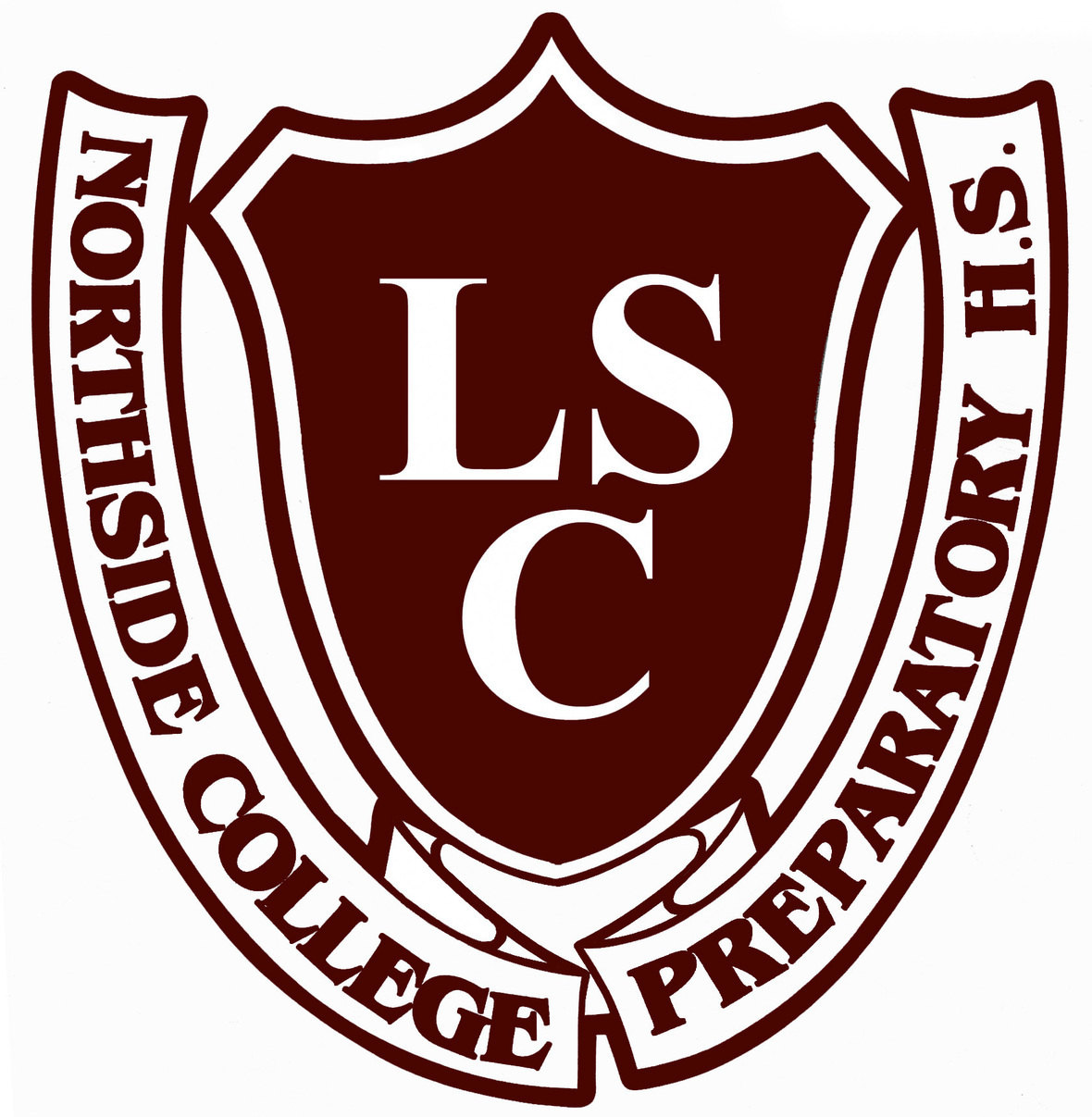# LSC Meeting

Tuesday, March 19
6:30 pm
Faculty Lounge

The LSC is going to start its annual evaluation of Ms. Mest and would like parent feedback. Please click HERE to complete the survey.

 table div table+table+table+table+table+table+table+table+table+table+table+table+table+table+table+table+table+table+table+table+table+table+table+table+table+table+table+table+table+table+table div table{width:100%;padding:0}table div table+table+table+table+table+table+table+table+table+table+table+table+table+table+table+table+table+table+table+table+table+table+table+table+table+table+table+table+table+table+table div table img{width:96.23%;padding:0;float:none}table div table+table+table+table+table+table+table+table+table+table+table+table+table+table+table+table+table+table+table+table+table+table+table+table+table+table+table+table+table+table+table div table td{width:100%;padding:0 1.88% 18px}/* styles */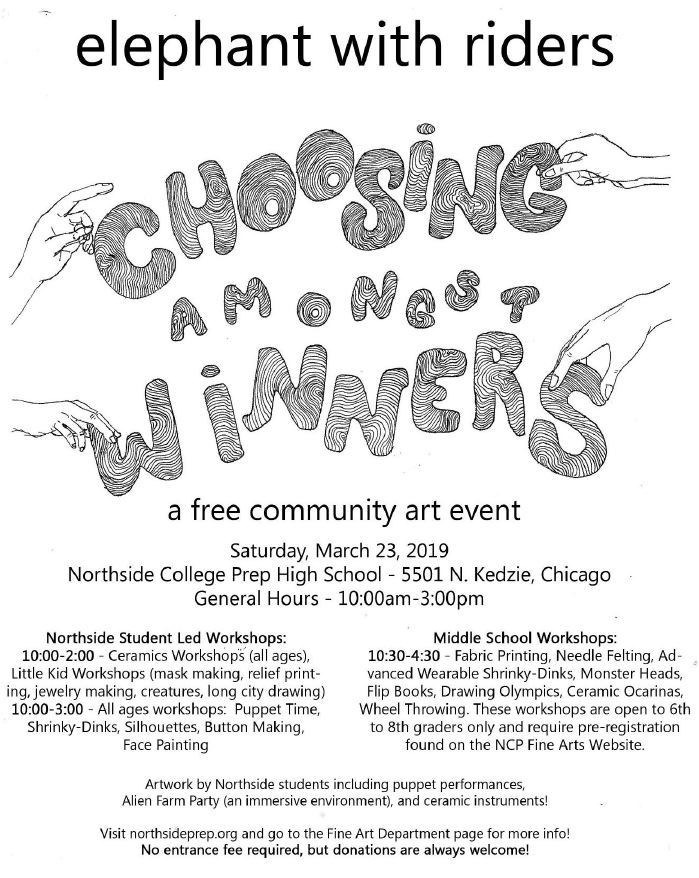# Elephant with Riders, Choosing Amongst Winners

## A Free Community Art Event at NCP

Saturday, March 23
10:00 am - 5:00 pm
Cafeteria and Art Wing

Free family art event for all ages featuring various art workshops taught by Northside students in ceramics, printmaking, shrinky dinks, puppet making, and more. Also featuring interactive art exhibits and special workshops for 6th-8th graders that require preregistration. See the Fine Arts Webpage for schedule of events and to preregister for the 6th-8th grade special workshops.

## Please Donate Materials

The art department is creating an immersive environment for Elephant with Riders and is looking for some items. If you have any of these items and are willing to donate them, please drop them off outside room 111 (art room). Contact Joanne Minyo (jminyo@cps.edu) with any questions. Thanks!

▪ Black or very dark fabric - large pieces only
▪ Extension Cords
 ▪ Black or very dark fabric - large pieces only
 ▪ Extension Cords
 table div table+table+table+table+table+table+table+table+table+table+table+table+table+table+table+table+table+table+table+table+table+table+table+table+table+table+table+table+table+table+table+table+table+table div table{width:100%;padding:0}table div table+table+table+table+table+table+table+table+table+table+table+table+table+table+table+table+table+table+table+table+table+table+table+table+table+table+table+table+table+table+table+table+table+table div table img{width:96.23%;padding:0;float:none}table div table+table+table+table+table+table+table+table+table+table+table+table+table+table+table+table+table+table+table+table+table+table+table+table+table+table+table+table+table+table+table+table+table+table div table td{width:100%;padding:0 1.88% 18px}/* styles */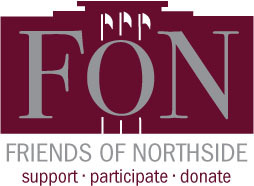# Friends of Northside Meeting

Tuesday, March 26
7:00 pm
Faculty Lounge

 table div table+table+table+table+table+table+table+table+table+table+table+table+table+table+table+table+table+table+table+table+table+table+table+table+table+table+table+table+table+table+table+table+table+table+table+table div table{width:100%;padding:0}table div table+table+table+table+table+table+table+table+table+table+table+table+table+table+table+table+table+table+table+table+table+table+table+table+table+table+table+table+table+table+table+table+table+table+table+table div table img{width:96.23%;padding:0;float:none}table div table+table+table+table+table+table+table+table+table+table+table+table+table+table+table+table+table+table+table+table+table+table+table+table+table+table+table+table+table+table+table+table+table+table+table+table div table td{width:100%;padding:0 1.88% 18px}/* styles */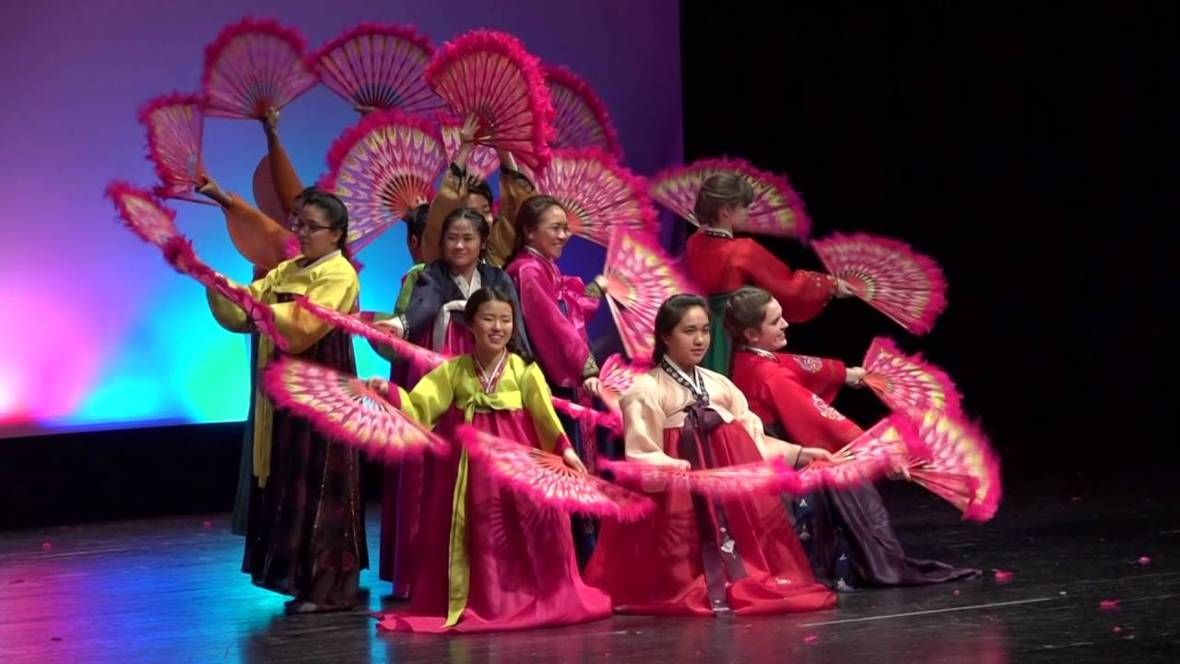# International Night 2019

## Dance Showcase

Friday, March 29, 7:00 pm
Saturday, March 30, 3:00 pm
Auditorium

I-Night is a cultural dance celebration of the many diverse groups across the school's community, and brings together the largest collection of student performers of any NCP event.

Tickets are \$10 (under 12 are free but still require a ticket). Please plan ahead as shows sell out. Tickets will be sold from March 18 - 22 during lunch blocks. No one will be admitted without a ticket so purchase for family, friends, and alumni. Since it is such a popular attended event, for the first two days of sales each student is capped at purchasing 10 tickets.
NO TICKETS WILL BE SOLD AT THE DOOR.

 table div table+table+table+table+table+table+table+table+table+table+table+table+table+table+table+table+table+table+table+table+table+table+table+table+table+table+table+table+table+table+table+table+table+table+table+table+table+table+table div table{width:100%;padding:0}table div table+table+table+table+table+table+table+table+table+table+table+table+table+table+table+table+table+table+table+table+table+table+table+table+table+table+table+table+table+table+table+table+table+table+table+table+table+table+table div table img{width:96.23%;padding:0;float:none}table div table+table+table+table+table+table+table+table+table+table+table+table+table+table+table+table+table+table+table+table+table+table+table+table+table+table+table+table+table+table+table+table+table+table+table+table+table+table+table div table td{width:100%;padding:0 1.88% 18px}/* styles */# SAVE THE DATE!

PRESENTS

## Parenting in the Age of Anxiety

May 2, 6:30 pm

FEATURING…

Dr. Debra Kissen

Dr. Debra Kissen is the Owner and Clinical Director of the Light On Anxiety Treatment Center. She specializes in Cognitive Behavioral Therapy (CBT) for anxiety and related disorders, is the author of the Panic Workbook for Teens and is an active contributor to the Huffington Post. Dr. Kissen will provide tips and strategies to assist with parenting in the age of anxiety

▪ How to tell the difference between every day stress and anxiety
▪ How to model effective coping skills for your child
▪ How to compassionately push your child forward to assist them in facing their fears
 ▪ How to tell the difference between every day stress and anxiety
 ▪ How to model effective coping skills for your child
 ▪ How to compassionately push your child forward to assist them in facing their fears

Look for additional details soon in the Northside Parents Facebook Page and at FriendsofNorthside.org

You can find additional information regarding the speaker and Light on Anxiety CBT Treatment Center at www.lightonanxiety.com

 table div table+table+table+table+table+table+table+table+table+table+table+table+table+table+table+table+table+table+table+table+table+table+table+table+table+table+table+table+table+table+table+table+table+table+table+table+table+table+table+table+table div table{width:100%;padding:0}table div table+table+table+table+table+table+table+table+table+table+table+table+table+table+table+table+table+table+table+table+table+table+table+table+table+table+table+table+table+table+table+table+table+table+table+table+table+table+table+table+table div table img{width:96.23%;padding:0;float:none}table div table+table+table+table+table+table+table+table+table+table+table+table+table+table+table+table+table+table+table+table+table+table+table+table+table+table+table+table+table+table+table+table+table+table+table+table+table+table+table+table+table div table td{width:100%;padding:0 1.88% 18px}/* styles */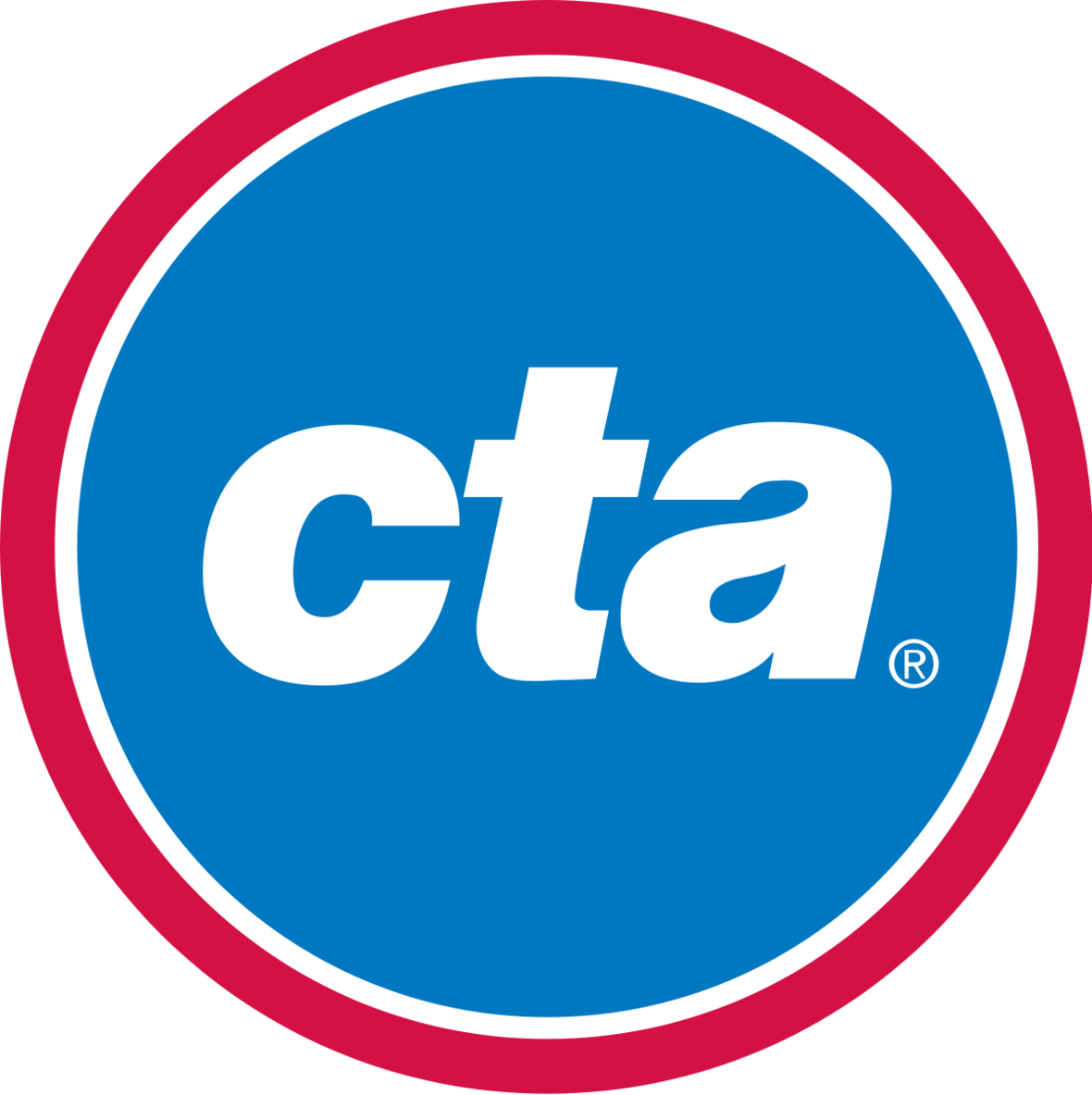# CTA Safety Presentation

Wednesday, May 22

A representative from CTA will present to NCP students about navigating and staying safe on public transportation. Please encourage your students to learn about the support and safety tips offered to ensure an enjoyable commute to and from school.

 table div table+table+table+table+table+table+table+table+table+table+table+table+table+table+table+table+table+table+table+table+table+table+table+table+table+table+table+table+table+table+table+table+table+table+table+table+table+table+table+table+table+table+table div table{width:100%;padding:0}table div table+table+table+table+table+table+table+table+table+table+table+table+table+table+table+table+table+table+table+table+table+table+table+table+table+table+table+table+table+table+table+table+table+table+table+table+table+table+table+table+table+table+table div table img{width:96.23%;padding:0;float:none}table div table+table+table+table+table+table+table+table+table+table+table+table+table+table+table+table+table+table+table+table+table+table+table+table+table+table+table+table+table+table+table+table+table+table+table+table+table+table+table+table+table+table+table div table td{width:100%;padding:0 1.88% 18px}/* styles */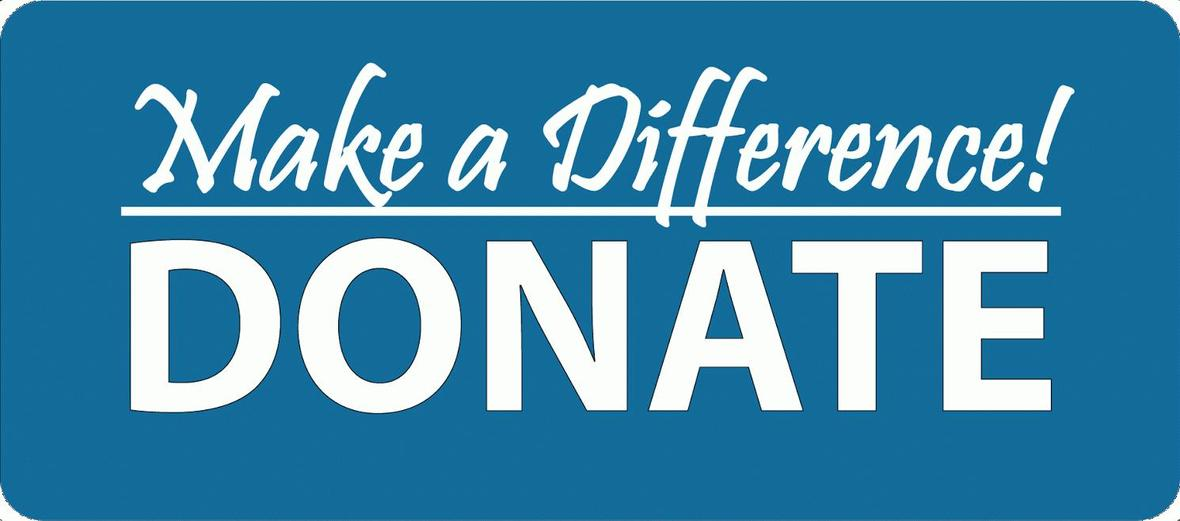# Student Resource Closet

## Donations Needed

Friends of Northside has a resource closet for our NCP students. We would like to fill it with school supplies, non-perishable food items, hygiene products, and clothing necessities.
All items can be brought into the counseling office and placed in the large bin marked “Student Supply Box.”

Please make sure all of the items are new and unopened. No used clothing please.

If you have any questions please contact Nancy Liljedahl at n.liljedahl@comcast.net.

Thanks for your help and support!

Supply List:

▪ Body lotion
▪ Reusable water bottles
▪ Girls shaving gel
▪ Individual snacks such as crackers, nuts, granola bars, or dried fruit
 ▪ Body lotion
 ▪ Reusable water bottles
 ▪ Girls shaving gel
 ▪ Individual snacks such as crackers, nuts, granola bars, or dried fruit

Please bring your items into the Counseling Office and put into the gray bin.

 table div table+table+table+table+table+table+table+table+table+table+table+table+table+table+table+table+table+table+table+table+table+table+table+table+table+table+table+table+table+table+table+table+table+table+table+table+table+table+table+table+table+table+table+table+table div table{width:100%;padding:0}table div table+table+table+table+table+table+table+table+table+table+table+table+table+table+table+table+table+table+table+table+table+table+table+table+table+table+table+table+table+table+table+table+table+table+table+table+table+table+table+table+table+table+table+table+table div table img{width:96.23%;padding:0;float:none}table div table+table+table+table+table+table+table+table+table+table+table+table+table+table+table+table+table+table+table+table+table+table+table+table+table+table+table+table+table+table+table+table+table+table+table+table+table+table+table+table+table+table+table+table+table div table td{width:100%;padding:0 1.88% 18px}/* styles */# Juniors - Class of 2020! Save the Dates!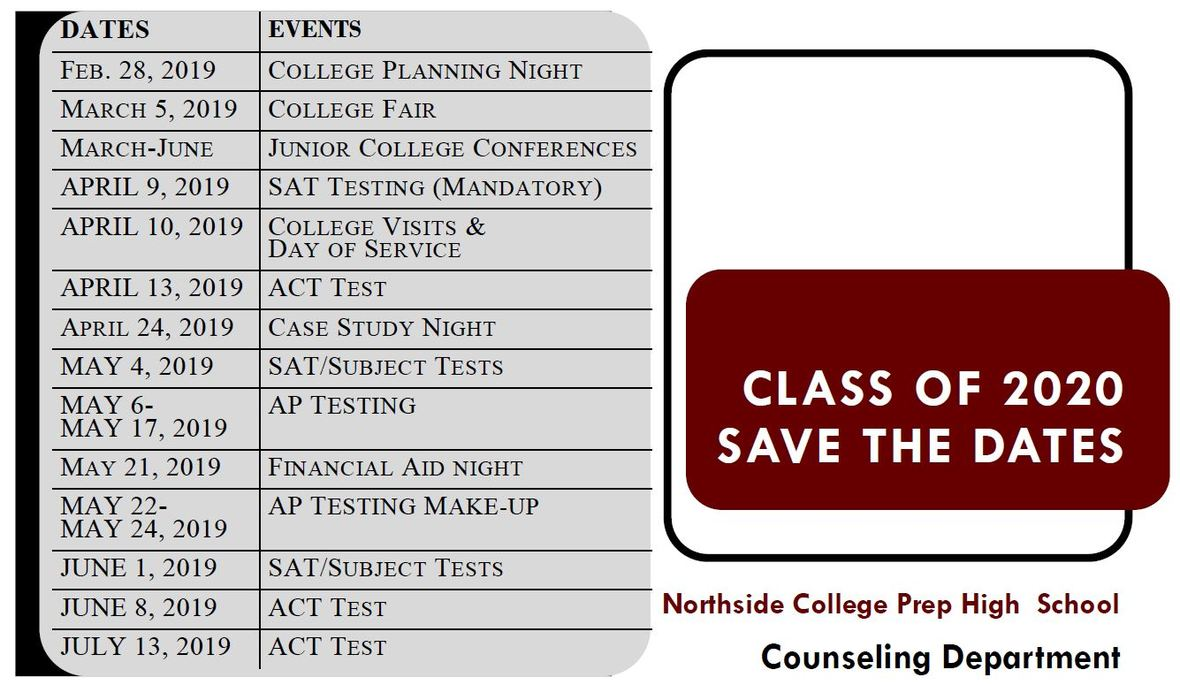table div table+table+table+table+table+table+table+table+table+table+table+table+table+table+table+table+table+table+table+table+table+table+table+table+table+table+table+table+table+table+table+table+table+table+table+table+table+table+table+table+table+table+table+table+table+table+table+table div table{width:100%;padding:0}table div table+table+table+table+table+table+table+table+table+table+table+table+table+table+table+table+table+table+table+table+table+table+table+table+table+table+table+table+table+table+table+table+table+table+table+table+table+table+table+table+table+table+table+table+table+table+table+table div table img{width:96.23%;padding:0;float:none}table div table+table+table+table+table+table+table+table+table+table+table+table+table+table+table+table+table+table+table+table+table+table+table+table+table+table+table+table+table+table+table+table+table+table+table+table+table+table+table+table+table+table+table+table+table+table+table+table div table td{width:100%;padding:0 1.88% 18px}/* styles */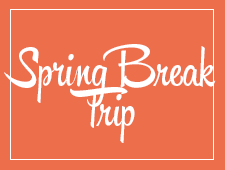# Travel this Spring with Northside

## Spring Break 2019 Trip to the Grand Canyon

Students will have a chance to connect with the majesty of our National Park system. We will spend 5 nights in the Grand Canyon camping, hiking, stargazing, and learning wilderness skills. Students will participate in a second-semester colloquium to prepare them for the trip. Trip sponsors and chaperones include Ms. Qazi, Ms. Schaldenbrand, and Mr. Barry Smith. Approximate cost: \$1,000 (includes flight, food, and lodging).

 table div table+table+table+table+table+table+table+table+table+table+table+table+table+table+table+table+table+table+table+table+table+table+table+table+table+table+table+table+table+table+table+table+table+table+table+table+table+table+table+table+table+table+table+table+table+table+table+table+table+table div table{width:100%;padding:0}table div table+table+table+table+table+table+table+table+table+table+table+table+table+table+table+table+table+table+table+table+table+table+table+table+table+table+table+table+table+table+table+table+table+table+table+table+table+table+table+table+table+table+table+table+table+table+table+table+table+table div table img{width:96.23%;padding:0;float:none}table div table+table+table+table+table+table+table+table+table+table+table+table+table+table+table+table+table+table+table+table+table+table+table+table+table+table+table+table+table+table+table+table+table+table+table+table+table+table+table+table+table+table+table+table+table+table+table+table+table+table div table td{width:100%;padding:0 1.88% 18px}/* styles */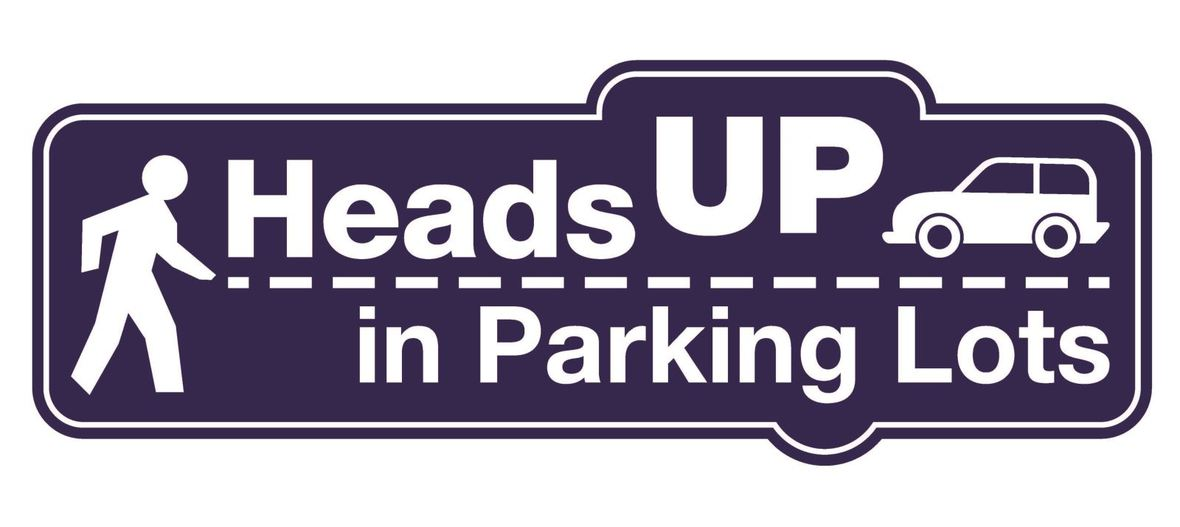# Parking Lot Safety and Procedures

Our NCP school parking lot is a busy place before and after school. Families dropping off and picking up students are asked to be mindful of both safety and courtesy. Please assist us by ensuring that traffic lanes stay clear during drop-off and pick-up times. Pulling over to the curb on the right as your student enters or exits your vehicle keeps students safe and traffic flow efficient.

Please remember that ALL VEHICLES MUST EXIT ONTO KEDZIE. You may enter the parking lot from Kedzie or Bryn Mawr, but regardless of where you enter, all cars must exit onto Kedzie. For the safety of our students and the rest of the Northside community, please do not exit onto Bryn Mawr.

 table div table+table+table+table+table+table+table+table+table+table+table+table+table+table+table+table+table+table+table+table+table+table+table+table+table+table+table+table+table+table+table+table+table+table+table+table+table+table+table+table+table+table+table+table+table+table+table+table+table+table+table+table+table div table{width:100%;padding:0}table div table+table+table+table+table+table+table+table+table+table+table+table+table+table+table+table+table+table+table+table+table+table+table+table+table+table+table+table+table+table+table+table+table+table+table+table+table+table+table+table+table+table+table+table+table+table+table+table+table+table+table+table+table div table img{width:96.23%;padding:0;float:none}table div table+table+table+table+table+table+table+table+table+table+table+table+table+table+table+table+table+table+table+table+table+table+table+table+table+table+table+table+table+table+table+table+table+table+table+table+table+table+table+table+table+table+table+table+table+table+table+table+table+table+table+table+table div table td{width:100%;padding:0 1.88% 18px}/* styles */# CPS Volunteer Requirements

According to CPS policy, anybody who is going to be in contact with students must be approved as a CPS volunteer. There are two levels of volunteers, Level 1 and Level 2.

## Level II Volunteers

(This process can be completed in a day & is required even of volunteers for Friends of Northside events)
A fingerprint criminal background check is not required for Level II Volunteers.

A Level II Volunteer is:

1. A parent providing supervised volunteer service in their child’s school or classroom for less than 10 hours/week;
2. Any individual providing volunteer service for less than 5 hours/week at a school where s/he does not have a child enrolled;
3. An individual providing incidental volunteer service with no ongoing individualized interaction with a student(s) including those who speak at a class/assembly, judge academic competitions, give musical performance, participate in the “Principal for a Day” program, job shadowing event or other one-time event provided where there is direct supervision of the activity/event by regular school employees;
4. A parent accompanying his/her child’s class on a one-day field trip or another type of extracurricular activity that does not involve an overnight stay; and/or
5. An individual providing volunteer service on projects/activities involving no or nominal contact with children (home-based volunteer activities, volunteers serving at Central or Network office).
 1 A parent providing supervised volunteer service in their child’s school or classroom for less than 10 hours/week;
 2 Any individual providing volunteer service for less than 5 hours/week at a school where s/he does not have a child enrolled;
 3 An individual providing incidental volunteer service with no ongoing individualized interaction with a student(s) including those who speak at a class/assembly, judge academic competitions, give musical performance, participate in the “Principal for a Day” program, job shadowing event or other one-time event provided where there is direct supervision of the activity/event by regular school employees;
 4 A parent accompanying his/her child’s class on a one-day field trip or another type of extracurricular activity that does not involve an overnight stay; and/or
 5 An individual providing volunteer service on projects/activities involving no or nominal contact with children (home-based volunteer activities, volunteers serving at Central or Network office).

## Level I Volunteers

(Please allow 8-12 weeks for the entire process)
A fingerprint criminal background check is required for all Level I Volunteers.

A Level I volunteer is:

1. A parent providing volunteer service in their child’s school or classroom for 10 or more hours/week;
2. An individual providing volunteer service for 5 or more hours/week at a school where s/he does not have a child enrolled; and/or
3. Any individual chaperoning an overnight school-sponsored trip, regardless of the hours/week that the volunteer serves (See also the Student Travel Policy).
 1 A parent providing volunteer service in their child’s school or classroom for 10 or more hours/week;
 2 An individual providing volunteer service for 5 or more hours/week at a school where s/he does not have a child enrolled; and/or
 3 Any individual chaperoning an overnight school-sponsored trip, regardless of the hours/week that the volunteer serves (See also the Student Travel Policy).

To become a volunteer, please visit CPS' Volunteer website and follow the instructions. There is also a link on Northside's website on the Parents page Northside Volunteers.

Assistant Principal Kaitlin O'Hare is Northside's Volunteer Coordinator. After applying to become a volunteer, you must visit the school and show Ms. O'Hare your ID, so she can approve you to move forward in the volunteer process. For Level II volunteers, that is the only step you must take. For Level I, you will receive further instructions from CPS.

 table div table+table+table+table+table+table+table+table+table+table+table+table+table+table+table+table+table+table+table+table+table+table+table+table+table+table+table+table+table+table+table+table+table+table+table+table+table+table+table+table+table+table+table+table+table+table+table+table+table+table+table+table+table+table+table div table{width:100%;padding:0}table div table+table+table+table+table+table+table+table+table+table+table+table+table+table+table+table+table+table+table+table+table+table+table+table+table+table+table+table+table+table+table+table+table+table+table+table+table+table+table+table+table+table+table+table+table+table+table+table+table+table+table+table+table+table+table div table img{width:96.23%;padding:0;float:none}table div table+table+table+table+table+table+table+table+table+table+table+table+table+table+table+table+table+table+table+table+table+table+table+table+table+table+table+table+table+table+table+table+table+table+table+table+table+table+table+table+table+table+table+table+table+table+table+table+table+table+table+table+table+table+table div table td{width:100%;padding:0 1.88% 18px}/* styles */table.module-55{width:94.34%;padding:0}table div table+table+table+table+table+table+table+table+table+table+table+table+table+table+table+table+table+table+table+table+table+table+table+table+table+table+table+table+table+table+table+table+table+table+table+table+table+table+table+table+table+table+table+table+table+table+table+table+table+table+table+table+table+table+table+table div table{width:94.34%;float:none;margin-left:auto;margin-right:auto;padding:0}table div table+table+table+table+table+table+table+table+table+table+table+table+table+table+table+table+table+table+table+table+table+table+table+table+table+table+table+table+table+table+table+table+table+table+table+table+table+table+table+table+table+table+table+table+table+table+table+table+table+table+table+table+table+table+table+table div table a{border:0 none;text-decoration:none}table div table+table+table+table+table+table+table+table+table+table+table+table+table+table+table+table+table+table+table+table+table+table+table+table+table+table+table+table+table+table+table+table+table+table+table+table+table+table+table+table+table+table+table+table+table+table+table+table+table+table+table+table+table+table+table+table div table img{width:100%!important;border:0 none;text-decoration:none}table div table+table+table+table+table+table+table+table+table+table+table+table+table+table+table+table+table+table+table+table+table+table+table+table+table+table+table+table+table+table+table+table+table+table+table+table+table+table+table+table+table+table+table+table+table+table+table+table+table+table+table+table+table+table+table+table div table td{width:100%;padding:0}/* styles */
 /* styles */ Check out The HoofBeat, Northside's online newspaper, to get NCP news from the student perspective!
 table div table+table+table+table+table+table+table+table+table+table+table+table+table+table+table+table+table+table+table+table+table+table+table+table+table+table+table+table+table+table+table+table+table+table+table+table+table+table+table+table+table+table+table+table+table+table+table+table+table+table+table+table+table+table+table+table+table+table div table{width:100%;padding:0}table div table+table+table+table+table+table+table+table+table+table+table+table+table+table+table+table+table+table+table+table+table+table+table+table+table+table+table+table+table+table+table+table+table+table+table+table+table+table+table+table+table+table+table+table+table+table+table+table+table+table+table+table+table+table+table+table+table+table div table img{width:96.23%;padding:0;float:none}table div table+table+table+table+table+table+table+table+table+table+table+table+table+table+table+table+table+table+table+table+table+table+table+table+table+table+table+table+table+table+table+table+table+table+table+table+table+table+table+table+table+table+table+table+table+table+table+table+table+table+table+table+table+table+table+table+table+table div table td{width:100%;padding:0 1.88% 18px}/* styles */table.module-58{width:57.17%;padding:0}table div table+table+table+table+table+table+table+table+table+table+table+table+table+table+table+table+table+table+table+table+table+table+table+table+table+table+table+table+table+table+table+table+table+table+table+table+table+table+table+table+table+table+table+table+table+table+table+table+table+table+table+table+table+table+table+table+table+table+table div table{width:57.17%;float:none;margin-left:auto;margin-right:auto;padding:0}table div table+table+table+table+table+table+table+table+table+table+table+table+table+table+table+table+table+table+table+table+table+table+table+table+table+table+table+table+table+table+table+table+table+table+table+table+table+table+table+table+table+table+table+table+table+table+table+table+table+table+table+table+table+table+table+table+table+table+table div table a{border:0 none;text-decoration:none}table div table+table+table+table+table+table+table+table+table+table+table+table+table+table+table+table+table+table+table+table+table+table+table+table+table+table+table+table+table+table+table+table+table+table+table+table+table+table+table+table+table+table+table+table+table+table+table+table+table+table+table+table+table+table+table+table+table+table+table div table img{width:100%!important;border:0 none;text-decoration:none}table div table+table+table+table+table+table+table+table+table+table+table+table+table+table+table+table+table+table+table+table+table+table+table+table+table+table+table+table+table+table+table+table+table+table+table+table+table+table+table+table+table+table+table+table+table+table+table+table+table+table+table+table+table+table+table+table+table+table+table div table td{width:100%;padding:0}/* styles */

# What is FAN?

FAN is Family Action Network, an organization which connects parents, educators, and professionals through collaborative programming that educates, inspires, and positively impacts the broader community. Nearly every week during the school year there will be a dynamic and important speaker, discussing the issues that interest you.

FAN events are free to all.
Click HERE to see upcoming events.
To visit FAN's website, click HERE.

 table div table+table+table+table+table+table+table+table+table+table+table+table+table+table+table+table+table+table+table+table+table+table+table+table+table+table+table+table+table+table+table+table+table+table+table+table+table+table+table+table+table+table+table+table+table+table+table+table+table+table+table+table+table+table+table+table+table+table+table+table+table div table{width:100%;padding:0}table div table+table+table+table+table+table+table+table+table+table+table+table+table+table+table+table+table+table+table+table+table+table+table+table+table+table+table+table+table+table+table+table+table+table+table+table+table+table+table+table+table+table+table+table+table+table+table+table+table+table+table+table+table+table+table+table+table+table+table+table+table div table img{width:96.23%;padding:0;float:none}table div table+table+table+table+table+table+table+table+table+table+table+table+table+table+table+table+table+table+table+table+table+table+table+table+table+table+table+table+table+table+table+table+table+table+table+table+table+table+table+table+table+table+table+table+table+table+table+table+table+table+table+table+table+table+table+table+table+table+table+table+table div table td{width:100%;padding:0 1.88% 18px}/* styles */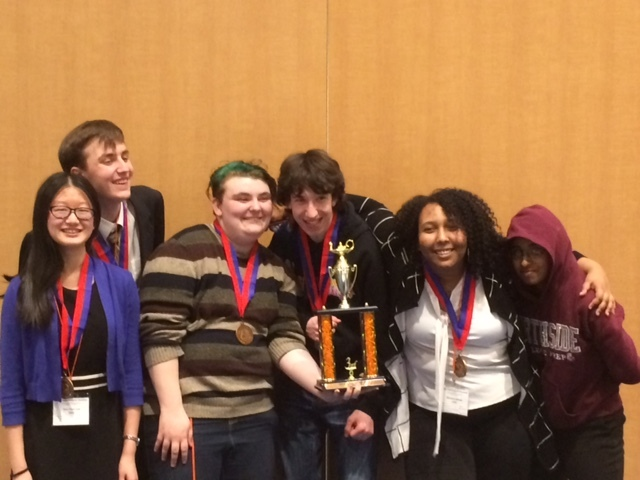# Northside's Academic Decathlon Team Places Third in State Competition, To Compete Again at Nationals

## Mackeda Sahelu wins Bronze in the Interview event!

Northside's Academic Decathlon team is one of only two teams in Illinois invited to the National competition in Bloomington, MN in April.
Congratulations to the team: Justin Schechter, Christine Alex, Tyler O'Brien, Mackeda Sahelu, Tian Chen Luo, Simon Carter and to Coach David Golden.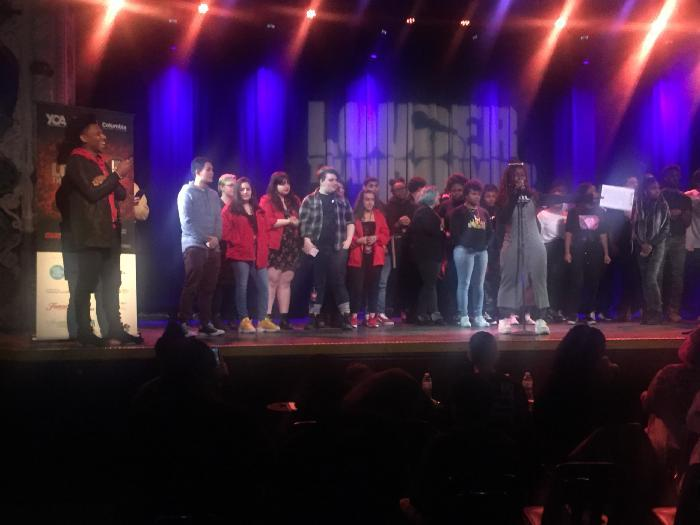# Northside's LTAB Team Finishes Season Strong

Northside's slam team made a fantastic run this Louder Than a Bomb season. With a few first time poets, as well as experienced veterans they finished 4th at semi-finals.

Emily Schultz (team captain)
Alexis Chilson (team captain)
Tyler O'Brien
Dhuha Wasfie
Llorenz Meliton
Sadia Haidari (first time poet)
Abigail Morales (first time poet)
Stephanie Burdin
Naseeba Yunusa (first time poet)
Albertas Kerelis (first time poet)
Lindsay Clark (junior poet)

# NCP's Debate Team has Strong Performance at JV/Novice state Tournament

The Northside debate team started off championship season with some momentum at the Illinois JV/Novice state title tournament at Maine East. Sophomores Evan Chen and Kyujin Derradji advanced to the state final match, losing a close 2-1 decision to Glenbrook North to fall just shy of a state championship and finish as state runner-up.

This is Northside's second year in a row appearing in a state title match, following Kyujin and Julia Torres' run to the novice state finals last year.

Kyujin and Evan compiled a 4-1 prelim record and picked up wins over New Trier, Oak Park/River Forest, and Walter Payton in elimination rounds on their way to the finals.

On the novice side, the freshmen teams of Mai-Linh Weller/Lillian Robinwhite and Jeannie Kim/Greg Schaefer also had strong runs, with both making it to the Sweet Sixteen of novice elimination rounds before bowing out to Lane Tech and Niles West, respectively.

The varsity state championship tournament is in Bloomington/Normal.

# Do you have news or team results to share with the Northside parent community?

## Click HERE to submit information.

 table div table+table+table+table+table+table+table+table+table+table+table+table+table+table+table+table+table+table+table+table+table+table+table+table+table+table+table+table+table+table+table+table+table+table+table+table+table+table+table+table+table+table+table+table+table+table+table+table+table+table+table+table+table+table+table+table+table+table+table+table+table+table+table+table+table+table+table+table+table div table{width:100%;padding:0}table div table+table+table+table+table+table+table+table+table+table+table+table+table+table+table+table+table+table+table+table+table+table+table+table+table+table+table+table+table+table+table+table+table+table+table+table+table+table+table+table+table+table+table+table+table+table+table+table+table+table+table+table+table+table+table+table+table+table+table+table+table+table+table+table+table+table+table+table+table div table img{width:96.23%;padding:0;float:none}table div table+table+table+table+table+table+table+table+table+table+table+table+table+table+table+table+table+table+table+table+table+table+table+table+table+table+table+table+table+table+table+table+table+table+table+table+table+table+table+table+table+table+table+table+table+table+table+table+table+table+table+table+table+table+table+table+table+table+table+table+table+table+table+table+table+table+table+table+table div table td{width:100%;padding:0 1.88% 18px}/* styles */# FON Connect

Friends of Northside uses a customer/constituent relationship management (CRM) system known as FON Connect that allows our parent volunteer organizations to work from one database.

FON Connect serves as the NCP Family Directory and allows users to review their donation history, update their contact information, register for upcoming events, and more. Each parent has a separate account. Transactions, including donations, are recorded in the account of the parent who initiated the transaction or donation.

If you’re new to FON Connect, you'll need to set up a login and password. To do so:

▪ Click HERE.
▪ Click on “Forgot Password?”
▪ Enter your email address.
▪ Check your email for the link to create a login name and password.
 ▪ Click HERE.
 ▪ Click on “Forgot Password?”
 ▪ Enter your email address.
 ▪ Check your email for the link to create a login name and password.

Once you have set up your FON Connect account, keep your login and password in a safe, convenient place because they will be your keys to the database throughout your years at Northside.

## Sign up and stay connected!

 table div table+table+table+table+table+table+table+table+table+table+table+table+table+table+table+table+table+table+table+table+table+table+table+table+table+table+table+table+table+table+table+table+table+table+table+table+table+table+table+table+table+table+table+table+table+table+table+table+table+table+table+table+table+table+table+table+table+table+table+table+table+table+table+table+table+table+table+table+table+table+table div table{width:100%;padding:0}table div table+table+table+table+table+table+table+table+table+table+table+table+table+table+table+table+table+table+table+table+table+table+table+table+table+table+table+table+table+table+table+table+table+table+table+table+table+table+table+table+table+table+table+table+table+table+table+table+table+table+table+table+table+table+table+table+table+table+table+table+table+table+table+table+table+table+table+table+table+table+table div table img{width:96.23%;padding:0;float:none}table div table+table+table+table+table+table+table+table+table+table+table+table+table+table+table+table+table+table+table+table+table+table+table+table+table+table+table+table+table+table+table+table+table+table+table+table+table+table+table+table+table+table+table+table+table+table+table+table+table+table+table+table+table+table+table+table+table+table+table+table+table+table+table+table+table+table+table+table+table+table+table div table td{width:100%;padding:0 1.88% 18px}/* styles */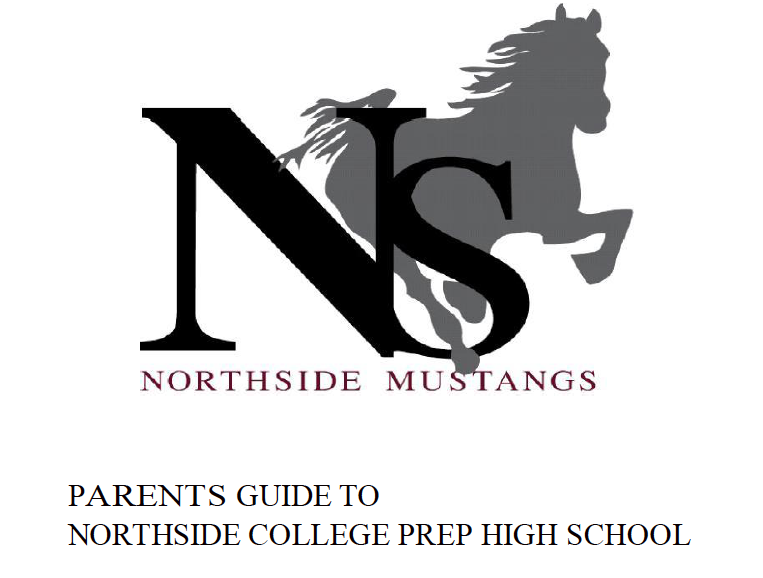# Navigating Northside

Navigating Northside, our parent handbook, is available on the school website under the Parents tab. Click HERE to access it directly.

 table div table+table+table+table+table+table+table+table+table+table+table+table+table+table+table+table+table+table+table+table+table+table+table+table+table+table+table+table+table+table+table+table+table+table+table+table+table+table+table+table+table+table+table+table+table+table+table+table+table+table+table+table+table+table+table+table+table+table+table+table+table+table+table+table+table+table+table+table+table+table+table+table+table div table{width:100%;padding:0}table div table+table+table+table+table+table+table+table+table+table+table+table+table+table+table+table+table+table+table+table+table+table+table+table+table+table+table+table+table+table+table+table+table+table+table+table+table+table+table+table+table+table+table+table+table+table+table+table+table+table+table+table+table+table+table+table+table+table+table+table+table+table+table+table+table+table+table+table+table+table+table+table+table div table img{width:96.23%;padding:0;float:none}table div table+table+table+table+table+table+table+table+table+table+table+table+table+table+table+table+table+table+table+table+table+table+table+table+table+table+table+table+table+table+table+table+table+table+table+table+table+table+table+table+table+table+table+table+table+table+table+table+table+table+table+table+table+table+table+table+table+table+table+table+table+table+table+table+table+table+table+table+table+table+table+table+table div table td{width:100%;padding:0 1.88% 18px}/* styles */# Spring Testing 2019 - SAT/PSAT

During the week of April 8, students in grades 9-11 will participate in city and statewide testing of the SAT/PSAT.

Tuesday, April 9
The SAT will be administered to students in 11th grade students..
Attendance day for 11th graders only; non-attendance day for 9th, 10th, and 12th graders

Wednesday, April 10
The PSAT will be administered to 9th and 10th grade students.

Attendance day for 9th and 10th graders only; non-attendance day for 11th and 12th graders

For 11th grade and 12th grade, there will be a series of optional college visits and service projects. Students will be asked to confirm participation.

# College Planning Night

## Junior Students and Parents,

Thank you for attending the Northside Prep, Junior College Planning Night on Thursday, March 14. The counseling team is looking forward to discussing your student's post-secondary plans during the upcoming College Conferences. For more details about scheduling your college conference contact your respective college counselor. To schedule a college conference with Ms. Medina, please contact Ms. Moore at mcmoore6@cps.edu.

If your family was unable to attend the College Planning Night, please visit our website to access the presentation and the College Planning Handbook (click HERE). Students, who did not attend the College Planning Night will be assigned to a Wednesday, X or Y-Block in the near future. Also, parents if you would like to access your Naviance log-in or update your address (click HERE) and submit your request to Ms. Fernandez at safernandez@cps.edu.

# CPS Scholarships and Non-NCP Events

For more information about general CPS scholarships and post-secondary events outside of Northside College Prep feel free to visit the NCP College Counseling Calendar HERE.

College Visits in March

Monday, March 18

▪ Colorado Mesa University, 9:48 am
▪ Hawaii Pacific University, 9:48 am
▪ Holy Cross College, 3:15 pm
 ▪ Colorado Mesa University, 9:48 am
 ▪ Hawaii Pacific University, 9:48 am
 ▪ Holy Cross College, 3:15 pm

Monday, March 25

▪ Roosevelt University, 11:36 am
 ▪ Roosevelt University, 11:36 am

Thursday, March 28

▪ University of Wisconsin, La Cross, 9:48 am
 ▪ University of Wisconsin, La Cross, 9:48 am

# College Counseling Calendar

For more details about the events listed below, visit the College Counseling Calendar on the Northside Prep Counseling website HERE.

Friday, March 22

▪ App Closes: Midwest Great Lakes Regional Scholarship
 ▪ App Closes: Midwest Great Lakes Regional Scholarship

Saturday, March 23

▪ App Closes: Urban Economics - Legacy Scholarship Chicago
 ▪ App Closes: Urban Economics - Legacy Scholarship Chicago

Monday, March 25

▪ App Closes: Computer Science Summer Institute for HBCU Students
 ▪ App Closes: Computer Science Summer Institute for HBCU Students

Wednesday, March 27

▪ App Closes: Cathay Bank Foundation Scholarship Program
 ▪ App Closes: Cathay Bank Foundation Scholarship Program

Friday, March 29

▪ App Closes: Guadalupe A. Reyes Fiesta Del Sol Scholarship
▪ App Closes: National African American Insurance Association (NAAIA) Chicago Chapter Scholarship
 ▪ App Closes: Guadalupe A. Reyes Fiesta Del Sol Scholarship
 ▪ App Closes: National African American Insurance Association (NAAIA) Chicago Chapter Scholarship

Sunday, March 31

▪ App Closes: Automotive Aftermarket Scholarships
▪ App Closes: Chicago Summer Business Institute (CSBI)
▪ App Closes: Chicago Urban League Scholarship
▪ App Closes: Cirkled In - "Live Forever" Scholarship
▪ App Closes: DePaul College Connect Summer Enrichment Program
▪ App Closes: Ewing-Jones Scholarship
▪ App Closes: Foster Care to Success Scholarship
▪ App Closes: Gread "Lefty" McKinnis Memorial Scholarship
▪ App Closes: IPLAC Educational Foundation Scholarship
▪ App Closes: Jack and Jill of America, Inc. Windy City Scholars
▪ App Closes: Monarch Awards Foundation Scholarship
 ▪ App Closes: Automotive Aftermarket Scholarships
 ▪ App Closes: Chicago Summer Business Institute (CSBI)
 ▪ App Closes: Chicago Urban League Scholarship
 ▪ App Closes: Cirkled In - "Live Forever" Scholarship
 ▪ App Closes: DePaul College Connect Summer Enrichment Program
 ▪ App Closes: Ewing-Jones Scholarship
 ▪ App Closes: Foster Care to Success Scholarship
 ▪ App Closes: Gread "Lefty" McKinnis Memorial Scholarship
 ▪ App Closes: IPLAC Educational Foundation Scholarship
 ▪ App Closes: Jack and Jill of America, Inc. Windy City Scholars
 ▪ App Closes: Monarch Awards Foundation Scholarship

# SAT Prep Course

Wednesdays through April 3, 2:00 - 5:00 pm
Bring sharpened pencils and a calculator for every class.

For more information, contact Monique Moore, Northside Prep College Counselor at mcmoore6@cps.edu or (773) 524-3954 x26811.# Naviance CCLR Live by Kaplan

## College, Career, and Life Readiness

Naviance users have free access to Kaplan's interactive live-streaming and on-demand programming. Click HERE to learn more.# NCP College Planning Handbook

This is a great resource that guides students through the entire college process. Click HERE to access a copy.

# NCP College Planning Additional Resources

Click on the links below to check out the additional resources on the Northside College Prep website:

 table div table+table+table+table+table+table+table+table+table+table+table+table+table+table+table+table+table+table+table+table+table+table+table+table+table+table+table+table+table+table+table+table+table+table+table+table+table+table+table+table+table+table+table+table+table+table+table+table+table+table+table+table+table+table+table+table+table+table+table+table+table+table+table+table+table+table+table+table+table+table+table+table+table+table+table+table+table+table+table+table+table+table+table+table div table{width:100%;padding:0}table div table+table+table+table+table+table+table+table+table+table+table+table+table+table+table+table+table+table+table+table+table+table+table+table+table+table+table+table+table+table+table+table+table+table+table+table+table+table+table+table+table+table+table+table+table+table+table+table+table+table+table+table+table+table+table+table+table+table+table+table+table+table+table+table+table+table+table+table+table+table+table+table+table+table+table+table+table+table+table+table+table+table+table+table div table img{width:96.23%;padding:0;float:none}table div table+table+table+table+table+table+table+table+table+table+table+table+table+table+table+table+table+table+table+table+table+table+table+table+table+table+table+table+table+table+table+table+table+table+table+table+table+table+table+table+table+table+table+table+table+table+table+table+table+table+table+table+table+table+table+table+table+table+table+table+table+table+table+table+table+table+table+table+table+table+table+table+table+table+table+table+table+table+table+table+table+table+table+table div table td{width:100%;padding:0 1.88% 18px}/* styles */# Thank you to our Individual and Corporate Sponsors

Friends of Northside and the 2019 Spring Benefit Committee would like to thank our Individual and Corporate Sponsors whose support was critical to making the event a financial success.

## Individual Sponsors

Platinum Sponsors

▪ Kristen & Paul Collier
▪ Julie Trester & Nick Newgarden
▪ Carol Anne & Bart Huff
▪ Jim Kosciolek & Jeanine O'Brien
▪ Janice Cody & Allen Wall
▪ Myra Wagner & Glen Spear
▪ Debra & Stephen Swan

Gold Sponsors

▪ Caryn & Charles Brissman
▪ Janice Maxwell & Kevin Carter
▪ Anne Marie Piazza-Cook & Rafael Cook
▪ Pamela Peters & Michael Hughes
▪ Dawn Bartholomew & Todd Maxwell
▪ Wendy & Matt Phillips
▪ Kim & George Ruhana
▪ Meg & Paul Salzetta
▪ Susan & John Schaefer
▪ Michele & Steven Sparks
▪ Elizabeth & George Phillips-Sorich

Silver Sponsors

▪ Izzi & Scott Baskin
▪ Kim Chhay & Edward Huang
▪ Pinki & Nehal Desai
▪ Jennifer & Tim Devine
▪ Holly & Paul Edlund
▪ Matthew & Lisa Farmer
▪ Anna Florek & Brian Carlson
▪ Dawn Zarembski & Mark Franke
▪ Nancy Liljedahl & Joel Friedman
▪ Linda & Ed Graziano
▪ Kimberly Chase & Joshua Harding
▪ Patricia Bayerlein & Michael Hoffman
▪ Kirsten Brandt & Steve Kibler
▪ Dianne & Thomas Malueg
▪ Kelly & Eric Mest
▪ Kamaljeet & Karna Murthy
▪ Art & Susan Nolan
▪ Ony Achufusi & Marta Olah
▪ Maria Maldonado & Esau Orduno
▪ Erin Menninger Klemm & Kurt Klemm
▪ Caroline & Ram Padmanabhan
▪ Lorna Propes & Ronald Himel
▪ Karen & Fabian Sanchez
▪ Matthew & Erika Schechter
▪ Irma & Peter Sodini
▪ Anne Suh & Alan Hauser
▪ Elizabeth & Brent Vincent
▪ Kelly & Michael Zehfuss

## Corporate Sponsorstable div table+table+table+table+table+table+table+table+table+table+table+table+table+table+table+table+table+table+table+table+table+table+table+table+table+table+table+table+table+table+table+table+table+table+table+table+table+table+table+table+table+table+table+table+table+table+table+table+table+table+table+table+table+table+table+table+table+table+table+table+table+table+table+table+table+table+table+table+table+table+table+table+table+table+table+table+table+table+table+table+table+table+table+table+table+table+table+table div table{width:100%;padding:0}table div table+table+table+table+table+table+table+table+table+table+table+table+table+table+table+table+table+table+table+table+table+table+table+table+table+table+table+table+table+table+table+table+table+table+table+table+table+table+table+table+table+table+table+table+table+table+table+table+table+table+table+table+table+table+table+table+table+table+table+table+table+table+table+table+table+table+table+table+table+table+table+table+table+table+table+table+table+table+table+table+table+table+table+table+table+table+table+table div table img{width:96.23%;padding:0;float:none}table div table+table+table+table+table+table+table+table+table+table+table+table+table+table+table+table+table+table+table+table+table+table+table+table+table+table+table+table+table+table+table+table+table+table+table+table+table+table+table+table+table+table+table+table+table+table+table+table+table+table+table+table+table+table+table+table+table+table+table+table+table+table+table+table+table+table+table+table+table+table+table+table+table+table+table+table+table+table+table+table+table+table+table+table+table+table+table+table div table td{width:100%;padding:0 1.88% 18px}/* styles */# Help Northside When You Shop Online

## You can help our school with no extra cost to you.

AmazonSmile is a simple and automatic way to support Northside while you shop.

Click HERE to read about AmazonSmile. Click HERE for detailed sign-up instructions. Skip the instructions and sign up HERE!

To choose Northside, please note that our AmazonSmile name is Friends of "North" "Side" (North Side - two words at AmazonSmile).

 table div table+table+table+table+table+table+table+table+table+table+table+table+table+table+table+table+table+table+table+table+table+table+table+table+table+table+table+table+table+table+table+table+table+table+table+table+table+table+table+table+table+table+table+table+table+table+table+table+table+table+table+table+table+table+table+table+table+table+table+table+table+table+table+table+table+table+table+table+table+table+table+table+table+table+table+table+table+table+table+table+table+table+table+table+table+table+table+table+table+table+table div table{width:100%;padding:0}table div table+table+table+table+table+table+table+table+table+table+table+table+table+table+table+table+table+table+table+table+table+table+table+table+table+table+table+table+table+table+table+table+table+table+table+table+table+table+table+table+table+table+table+table+table+table+table+table+table+table+table+table+table+table+table+table+table+table+table+table+table+table+table+table+table+table+table+table+table+table+table+table+table+table+table+table+table+table+table+table+table+table+table+table+table+table+table+table+table+table+table div table img{width:96.23%;padding:0;float:none}table div table+table+table+table+table+table+table+table+table+table+table+table+table+table+table+table+table+table+table+table+table+table+table+table+table+table+table+table+table+table+table+table+table+table+table+table+table+table+table+table+table+table+table+table+table+table+table+table+table+table+table+table+table+table+table+table+table+table+table+table+table+table+table+table+table+table+table+table+table+table+table+table+table+table+table+table+table+table+table+table+table+table+table+table+table+table+table+table+table+table+table div table td{width:100%;padding:0 1.88% 18px}/* styles */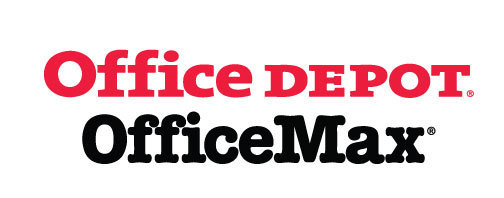# Give Back to Northside

Purchase school and office supplies at Office Depot and OfficeMax, and Northside can receive 5% back in credits or FREE supplies. Simply provide our school ID 70031171 at checkout, online or in stores. There’s no cost to you!

 table div table+table+table+table+table+table+table+table+table+table+table+table+table+table+table+table+table+table+table+table+table+table+table+table+table+table+table+table+table+table+table+table+table+table+table+table+table+table+table+table+table+table+table+table+table+table+table+table+table+table+table+table+table+table+table+table+table+table+table+table+table+table+table+table+table+table+table+table+table+table+table+table+table+table+table+table+table+table+table+table+table+table+table+table+table+table+table+table+table+table+table+table+table+table div table{width:100%;padding:0}table div table+table+table+table+table+table+table+table+table+table+table+table+table+table+table+table+table+table+table+table+table+table+table+table+table+table+table+table+table+table+table+table+table+table+table+table+table+table+table+table+table+table+table+table+table+table+table+table+table+table+table+table+table+table+table+table+table+table+table+table+table+table+table+table+table+table+table+table+table+table+table+table+table+table+table+table+table+table+table+table+table+table+table+table+table+table+table+table+table+table+table+table+table+table div table img{width:96.23%;padding:0;float:none}table div table+table+table+table+table+table+table+table+table+table+table+table+table+table+table+table+table+table+table+table+table+table+table+table+table+table+table+table+table+table+table+table+table+table+table+table+table+table+table+table+table+table+table+table+table+table+table+table+table+table+table+table+table+table+table+table+table+table+table+table+table+table+table+table+table+table+table+table+table+table+table+table+table+table+table+table+table+table+table+table+table+table+table+table+table+table+table+table+table+table+table+table+table+table div table td{width:100%;padding:0 1.88% 18px}/* styles */# Follow Us on Social Media

▪ NCP's Official Facebook Page
▪ Northside Prep Parents' Facebook Page, a closed group for parents, by parents, to share their knowledge of Northside
▪ NCP's Twitter Page
▪ Friends of Northside Facebook Page, for news everyone can view
 ▪ Northside Prep Parents' Facebook Page, a closed group for parents, by parents, to share their knowledge of Northside
 ▪ Friends of Northside Facebook Page, for news everyone can view
 table div table+table+table+table+table+table+table+table+table+table+table+table+table+table+table+table+table+table+table+table+table+table+table+table+table+table+table+table+table+table+table+table+table+table+table+table+table+table+table+table+table+table+table+table+table+table+table+table+table+table+table+table+table+table+table+table+table+table+table+table+table+table+table+table+table+table+table+table+table+table+table+table+table+table+table+table+table+table+table+table+table+table+table+table+table+table+table+table+table+table+table+table+table+table+table+table div table{width:100%;padding:0}table div table+table+table+table+table+table+table+table+table+table+table+table+table+table+table+table+table+table+table+table+table+table+table+table+table+table+table+table+table+table+table+table+table+table+table+table+table+table+table+table+table+table+table+table+table+table+table+table+table+table+table+table+table+table+table+table+table+table+table+table+table+table+table+table+table+table+table+table+table+table+table+table+table+table+table+table+table+table+table+table+table+table+table+table+table+table+table+table+table+table+table+table+table+table+table+table div table img{width:96.23%;padding:0;float:none}table div table+table+table+table+table+table+table+table+table+table+table+table+table+table+table+table+table+table+table+table+table+table+table+table+table+table+table+table+table+table+table+table+table+table+table+table+table+table+table+table+table+table+table+table+table+table+table+table+table+table+table+table+table+table+table+table+table+table+table+table+table+table+table+table+table+table+table+table+table+table+table+table+table+table+table+table+table+table+table+table+table+table+table+table+table+table+table+table+table+table+table+table+table+table+table+table div table td{width:100%;padding:0 1.88% 18px}/* styles */To view this message in your browser, click HERE.

Seniors, click HERE for the Class of 2019 web page.

Northside’s website can be found HERE.

Friends of Northside's website can be found HERE.

To see all volunteer opportunities on one page, click HERE.

To ensure delivery of This Week at Northside, please add Thisweek@friendsofnorthside.org to your address book.

Please do not reply to this e-mail. Send your questions or comments to Friends of Northside.

# Do you have news or team results to share with the Northside parent community?

## Click HERE to submit information.

 table div table+table+table+table+table+table+table+table+table+table+table+table+table+table+table+table+table+table+table+table+table+table+table+table+table+table+table+table+table+table+table+table+table+table+table+table+table+table+table+table+table+table+table+table+table+table+table+table+table+table+table+table+table+table+table+table+table+table+table+table+table+table+table+table+table+table+table+table+table+table+table+table+table+table+table+table+table+table+table+table+table+table+table+table+table+table+table+table+table+table+table+table+table+table+table+table+table+table div table{width:100%;padding:0}table div table+table+table+table+table+table+table+table+table+table+table+table+table+table+table+table+table+table+table+table+table+table+table+table+table+table+table+table+table+table+table+table+table+table+table+table+table+table+table+table+table+table+table+table+table+table+table+table+table+table+table+table+table+table+table+table+table+table+table+table+table+table+table+table+table+table+table+table+table+table+table+table+table+table+table+table+table+table+table+table+table+table+table+table+table+table+table+table+table+table+table+table+table+table+table+table+table+table div table img{width:96.23%;padding:0;float:none}table div table+table+table+table+table+table+table+table+table+table+table+table+table+table+table+table+table+table+table+table+table+table+table+table+table+table+table+table+table+table+table+table+table+table+table+table+table+table+table+table+table+table+table+table+table+table+table+table+table+table+table+table+table+table+table+table+table+table+table+table+table+table+table+table+table+table+table+table+table+table+table+table+table+table+table+table+table+table+table+table+table+table+table+table+table+table+table+table+table+table+table+table+table+table+table+table+table+table div table td{width:100%;padding:0 1.88% 18px}/* styles *//* styles */